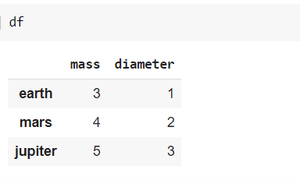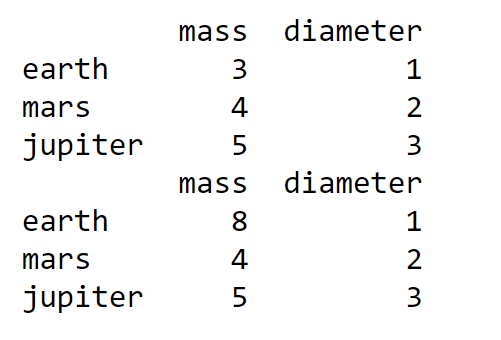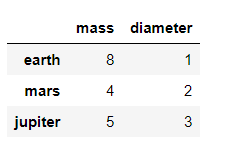Open in App
Not now

# Python Pandas – DataFrame.copy() function

• Difficulty Level : Basic
• Last Updated : 26 Nov, 2020

Python is a great language for doing data analysis, primarily because of the fantastic ecosystem of data-centric python packages. Pandas is one of those packages and makes importing and analyzing data much easier.

There are many ways to copy DataFrame in pandas. The first way is a simple way of assigning a dataframe object to a variable, but this has some drawbacks.

Syntax: DataFrame.copy(deep=True)

When deep=True (default), a new object will be created with a copy of the calling object’s data and indices. Modifications to the data or indices of the copy will not be reflected in the original object (see notes below).

When deep=False, a new object will be created without copying the calling object’s data or index (only references to the data and index are copied). Any changes to the data of the original will be reflected in the shallow copy (and vice versa).

Step 1) Let us first make a dummy data frame, which we will use for our illustration

Step 2) Assign that dataframe object to a variable

Step 3) Make changes in the original dataframe to see if there is any difference in copied variable

## Python3

 `import` `pandas as pd ` ` `  `#Create Series ` `s ``=` `pd.Series([``3``,``4``,``5``],[``'earth'``,``'mars'``,``'jupiter'``]) ` `k ``=` `pd.Series([``1``,``2``,``3``],[``'earth'``,``'mars'``,``'jupiter'``]) ` ` `  `#Create DataFrame df from two series ` `df ``=` `pd.DataFrame({``'mass'``:s,``'diameter'``:k}) ` ` `  `df `

Output:Dummy DataFrame df

Now, let’s assign the dataframe df to a variable and perform changes:

## Python3

 `#Assign df to variable_copy ` `variable_copy ``=` `df ` ` `  `print``(variable_copy)  ` `#Update the value of mass of earth in original dataframe ` `df[``'mass'``][``'earth'``]``=``8` ` `  `print``(variable_copy)`

Output:Here, we can see that if we change the values in the original dataframe, then the data in the copied variable also changes. To overcome this, we use DataFrame.copy()

Let us see this, with examples when deep=True(default ):

## Python3

 `res ``=` `df.copy(deep``=``True``) ` `print``(res)`

Output:My Personal Notes arrow_drop_up
Related Articles